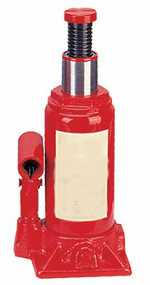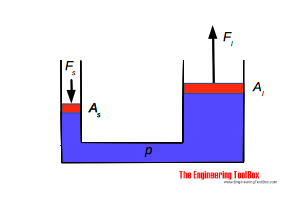Engineering ToolBox - Resources, Tools and Basic Information for Engineering and Design of Technical Applications!

# Hydraulic Force vs. Pascal's Law

## Pascal's law and the hydraulic force acting in fluids.

Pascal's Laws relates to pressures in incompressible fluids - liquids.

• if the weight of a fluid is neglected the pressure throughout an enclosed volume will be the same
• the static pressure in a fluid acts equally in all directions
• the static pressure acts at right angles to any surface in contact with the fluid

### Example - Pressure in a Hydraulic Cylinder

The pressure of 2000 Pa in an hydraulic cylinder acts equally on all surfaces. The force on a piston with area 0.1 m 2 can be calculated

F = p A                              (1)

where

F = force (N)

p = pressure (Pa, N/m 2 )

A = area (m 2 )

or with values

F = (2000 Pa) (0.1 m 2 )

= 200 (N)

### Example - Force in a Hydraulic JackThe pressure acting on both pistons in a hydraulic jack is equal.The force equation for the small cylinder:

F s = p A s (2)

where

F s = force acting on the piston in the small cylinder (N)

A s = area of small cylinder (m 2 )

p = pressure in small and large cylinder  (Pa, N/m 2 )

The force equation for the large cylinder:

F l = p A l (2b)

where

F l = force acting on the piston in the large cylinder (N)

A l = area of large cylinder (m 2 )

p = pressure in small and large cylinder (Pa, N/m 2 )

(2) and (2b) can be combined to

F s / A s = F l / A l (2c)

or

F s = F l A s / A l (2d)

The equation indicates that the effort force required in the small cylinder to lift a load on the large cylinder depends on the area ratio between the small and the large cylinder - the effort force can be reduced by reducing the small cylinder area compared to the large cylinder area.

### Related Mobile Apps from The Engineering ToolBox- free apps for offline use on mobile devices.

### A Hydraulic Jack Lifting a Car

The back end (half the weight) of a car of mass 2000 kg is lifted by an hydraulic jack where the A s / A l ratio is 0.1 (the area of the large cylinder is 10 times the area of the small cylinder).

The force - weight - acting on the large cylinder can be calculated with Newton's Second Law:

F l = m a

where

m = mass (kg)

a = acceleration of gravity (m/s 2 )

or

F l = 1/2 (2000 kg) (9.81 m/s 2 )

= 9810 (N)

The force acting on the small cylinder in the jack can be calculated with (2d)

F s = (9810 N) 0.1

= 981 (N)

## Related Topics

• ### Fluid Mechanics

The study of fluids - liquids and gases. Involving velocity, pressure, density and temperature as functions of space and time.
• ### Gases and Compressed Air

Air, LNG, LPG and other common gas properties, pipeline capacities, sizing of relief valves.

## Related Documents

• ### Hydraulic Force vs. Pressure and Cylinder Size

Calculate hydraulic cylinder force.
• ### Hydrostatic Pressure vs. Depth

Depth and hydrostatic pressure.
• ### Pressure

Introduction to pressure - online pressure units converter.

## Search

Search is the most efficient way to navigate the Engineering ToolBox.

## Engineering ToolBox - SketchUp Extension - Online 3D modeling!

Add standard and customized parametric components - like flange beams, lumbers, piping, stairs and more - to your Sketchup model with the Engineering ToolBox - SketchUp Extension - enabled for use with older versions of the amazing SketchUp Make and the newer "up to date" SketchUp Pro . Add the Engineering ToolBox extension to your SketchUp Make/Pro from the Extension Warehouse !

We don't collect information from our users. More about

## Citation

• The Engineering ToolBox (2008). Hydraulic Force vs. Pascal's Law. [online] Available at: https://www.engineeringtoolbox.com/pascal-laws-d_1274.html [Accessed Day Month Year].

Modify the access date according your visit.

12.8.9